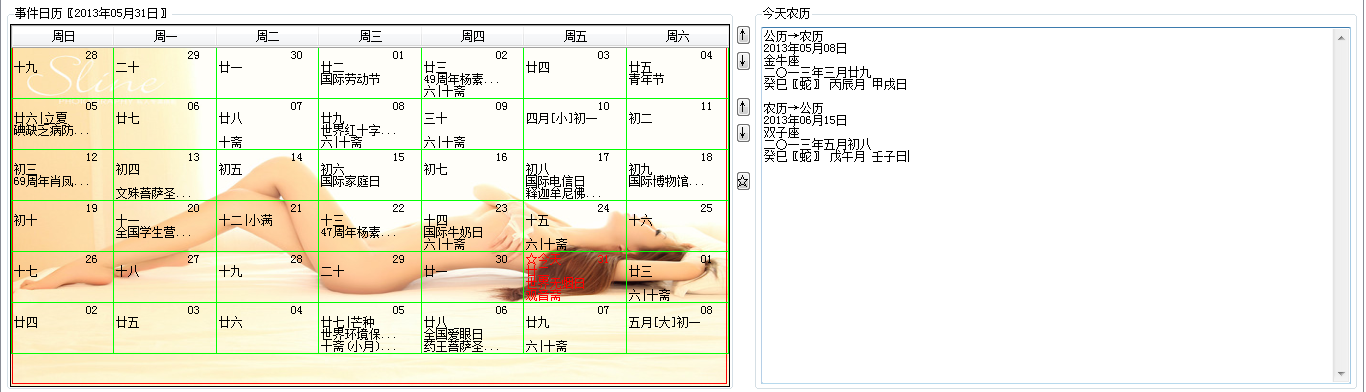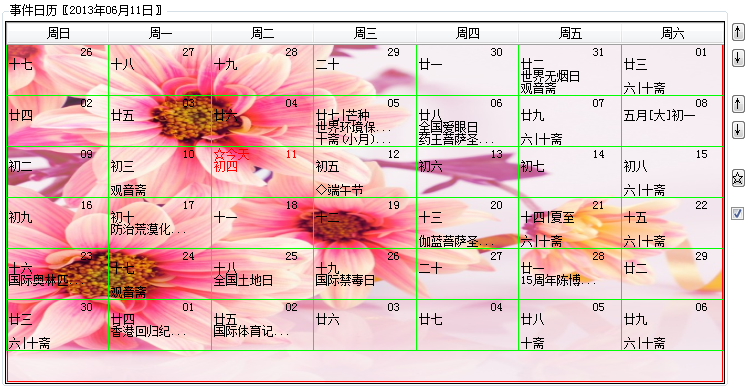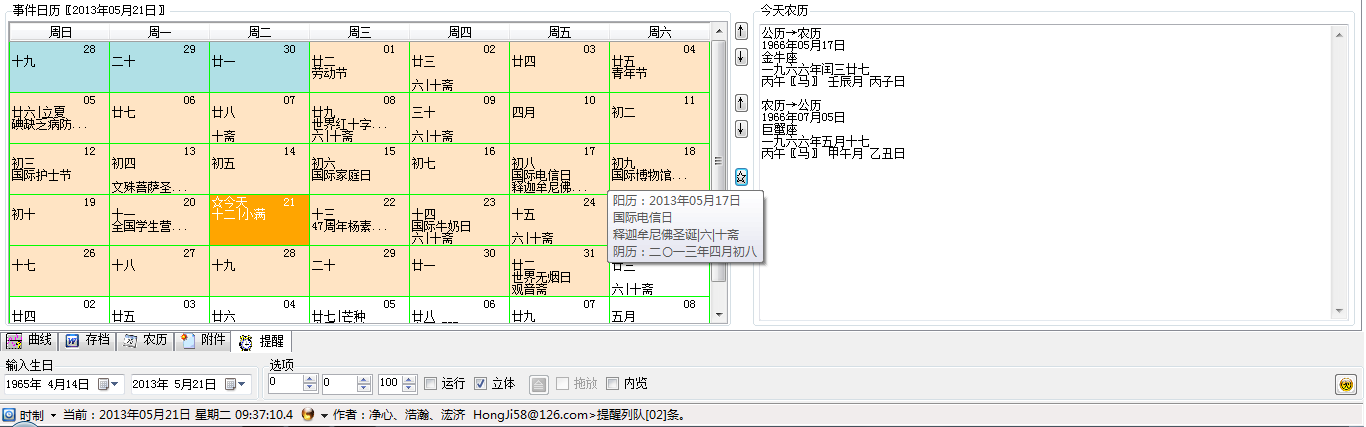# 手把手一步步用DataGridView 控件编写属于自己的日历string[] 日历列 = new string[] { "周日", "周一", "周二", "周三", "周四", "周五", "周六" };
foreach (string 元素 in 日历列)
{
DataGridViewTextBoxColumn 列 = new DataGridViewTextBoxColumn();
}


事件日历.Rows.Add(6);

string[] 日历列 = new string[] { "周日", "周一", "周二", "周三", "周四", "周五", "周六" };
foreach (string 元素 in 日历列)
{
DataGridViewTextBoxColumn 列 = new DataGridViewTextBoxColumn();
}



int 计数 = 0;
for (int 行 = 0; 行 < 事件日历.RowCount; 行++)
{
for (int 列 = 0; 列 < 事件日历.ColumnCount; 列++)
{
计数++;
if (计数 > 31) break;
事件日历.Rows[行].Cells[列].Value = 计数.ToString("00");
}
}


string[] 日历列 = new string[] { "周日", "周一", "周二", "周三", "周四", "周五", "周六" };
foreach (string 元素 in 日历列)
{
DataGridViewTextBoxColumn 列 = new DataGridViewTextBoxColumn();
}

int 计数 = 0;
for (int 行 = 0; 行 < 事件日历.RowCount; 行++)
{
for (int 列 = 0; 列 < 事件日历.ColumnCount; 列++)
{
计数++;
if (计数 > 31) break;
事件日历.Rows[行].Cells[列].Value = 计数.ToString("00");
}
}int 星期值 = Convert.ToInt16(DateTime.Parse(DateTime.Now.ToString("yyyy年MM月01日")).DayOfWeek);
int 月天数 = DateTime.DaysInMonth(DateTime.Now.Year, DateTime.Now.Month);


string[] 日历列 = new string[] { "周日", "周一", "周二", "周三", "周四", "周五", "周六" };
foreach (string 元素 in 日历列)
{
DataGridViewTextBoxColumn 列 = new DataGridViewTextBoxColumn();
}

int 计数 = 0;
int 星期值 = Convert.ToInt16(DateTime.Parse(DateTime.Now.ToString("yyyy年MM月01日")).DayOfWeek);
int 月天数 = DateTime.DaysInMonth(DateTime.Now.Year, DateTime.Now.Month);
for (int 行 = 0; 行 < 事件日历.RowCount; 行++)
{

if (行 > 0) 星期值 = 0;
for (int 列 = 星期值; 列 < 事件日历.ColumnCount; 列++)
{
计数++;
if (计数 > 月天数) break;
事件日历.Rows[行].Cells[列].Value = 计数.ToString("00");

}
}if (计数 > 月天数) 计数 = 1;

string[] 日历列 = new string[] { "周日", "周一", "周二", "周三", "周四", "周五", "周六" };
foreach (string 元素 in 日历列)
{
DataGridViewTextBoxColumn 列 = new DataGridViewTextBoxColumn();
}

int 计数 = 0;
int 星期值 = Convert.ToInt16(DateTime.Parse(DateTime.Now.ToString("yyyy年MM月01日")).DayOfWeek);
int 月天数 = DateTime.DaysInMonth(DateTime.Now.Year, DateTime.Now.Month);
for (int 行 = 0; 行 < 事件日历.RowCount; 行++)
{
if (行 > 0) 星期值 = 0;
for (int 列 = 星期值; 列 < 事件日历.ColumnCount; 列++)
{
计数++;
if (计数 > 月天数) 计数 = 1;
事件日历.Rows[行].Cells[列].Value = 计数.ToString("00");

}
}


int 上月天数 = DateTime.DaysInMonth(DateTime.Now.Year, DateTime.Now.Month - 1);
for (int 列 = 事件日历.ColumnCount - 1; 列 >= 0; 列--)
{
if (事件日历.Rows.Cells[列].Value == null)
{
事件日历.Rows.Cells[列].Value = 上月天数.ToString("00");
上月天数--;
}
}


string[] 日历列 = new string[] { "周日", "周一", "周二", "周三", "周四", "周五", "周六" };
foreach (string 元素 in 日历列)
{
DataGridViewTextBoxColumn 列 = new DataGridViewTextBoxColumn();
}

int 计数 = 0;
int 星期值 = Convert.ToInt16(DateTime.Parse(DateTime.Now.ToString("yyyy年MM月01日")).DayOfWeek);
int 月天数 = DateTime.DaysInMonth(DateTime.Now.Year, DateTime.Now.Month);
for (int 行 = 0; 行 < 事件日历.RowCount; 行++)
{
if (行 > 0) 星期值 = 0;
for (int 列 = 星期值; 列 < 事件日历.ColumnCount; 列++)
{
计数++;
if (计数 > 月天数) 计数 = 1;
事件日历.Rows[行].Cells[列].Value = 计数.ToString("00");
}
}
int 上月天数 = DateTime.DaysInMonth(DateTime.Now.Year, DateTime.Now.Month - 1);
for (int 列 = 事件日历.ColumnCount - 1; 列 >= 0; 列--)
{
if (事件日历.Rows.Cells[列].Value == null)
{
事件日历.Rows.Cells[列].Value = 上月天数.ToString("00");
上月天数--;
}
}

事件日历.CurrentCell = 事件日历.Rows[行].Cells[列];

string[] 日历列 = new string[] { "周日", "周一", "周二", "周三", "周四", "周五", "周六" };
foreach (string 元素 in 日历列)
{
DataGridViewTextBoxColumn 列 = new DataGridViewTextBoxColumn();
}

int 计数 = 0;
int 星期值 = Convert.ToInt16(DateTime.Parse(DateTime.Now.ToString("yyyy年MM月01日")).DayOfWeek);
int 月天数 = DateTime.DaysInMonth(DateTime.Now.Year, DateTime.Now.Month);
for (int 行 = 0; 行 < 事件日历.RowCount; 行++)
{
if (行 > 0) 星期值 = 0;
for (int 列 = 星期值; 列 < 事件日历.ColumnCount; 列++)
{
计数++;
if (计数 > 月天数) 计数 = 1;
事件日历.Rows[行].Cells[列].Value = 计数.ToString("00");
if(计数 == DateTime.Now.Day) 事件日历.CurrentCell = 事件日历.Rows[行].Cells[列];
}
}
int 上月天数 = DateTime.DaysInMonth(DateTime.Now.Year, DateTime.Now.Month - 1);
for (int 列 = 事件日历.ColumnCount - 1; 列 >= 0; 列--)
{
if (事件日历.Rows.Cells[列].Value == null)
{
事件日历.Rows.Cells[列].Value = 上月天数.ToString("00");
上月天数--;
}
}int 上月天数 = DateTime.DaysInMonth(DateTime.Now.Year, DateTime.Now.Month - 1);

for (int 列 = 星期值 - 1; 列 >= 0; 列--)
{
事件日历.Rows.Cells[列].Value = 上月天数.ToString("00");
上月天数--;
}


internal enum 天干 { 南無阿弥陀佛, 甲, 乙, 丙, 丁, 戊, 己, 庚, 辛, 壬, 癸 }
internal enum 地支 { 南無释迦牟尼佛, 子, 丑, 寅, 卯, 辰, 巳, 午, 未, 申, 酉, 戌, 亥 }
internal enum 生肖 { 南無阿弥陀佛, 鼠, 牛, 虎, 兔, 龙, 蛇, 马, 羊, 猴, 鸡, 狗, 猪 }
internal enum 中月 { 南無阿弥陀佛, 正, 二, 三, 四, 五, 六, 七, 八, 九, 十, 冬, 腊 }
internal enum 中数 { 〇, 一, 二, 三, 四, 五, 六, 七, 八, 九 }

ChineseLunisolarCalendar 微软农历 = new ChineseLunisolarCalendar();
int 甲子60数 = 微软农历.GetSexagenaryYear(DateTime.Now);
string 年干支 = ((天干)(微软农历.GetCelestialStem(甲子60数))).ToString()
+ ((地支)(微软农历.GetTerrestrialBranch(甲子60数))).ToString()
+ "〖" + ((生肖)(微软农历.GetTerrestrialBranch(甲子60数))).ToString() + "〗";
string 中文年 = "";
foreach (char 元素 in 微软农历.GetYear(DateTime.Now).ToString())
{ 中文年 += ((中数)(int.Parse(元素.ToString()))).ToString(); } 中文年 += "年";
string 中农历月 = "";
if (微软农历.IsLeapYear(微软农历.GetYear(DateTime.Now)))
if (微软农历.GetMonth(DateTime.Now) >= 微软农历.GetLeapMonth(微软农历.GetYear(DateTime.Now)))
中农历月 = (微软农历.GetMonth(DateTime.Now) == 微软农历.GetLeapMonth(微软农历.GetYear(DateTime.Now))) ?
"闰" + ((中月)微软农历.GetLeapMonth(微软农历.GetYear(DateTime.Now)) - 1).ToString() :
((中月)微软农历.GetMonth(DateTime.Now) - 1).ToString() + "月";
else
中农历月 = ((中月)微软农历.GetMonth(new DateTime(2013, 5, 17))).ToString() + "月";
int 农历日 = 微软农历.GetDayOfMonth(new DateTime(2013, 5, 17));
string 中农历日 = "";
if (农历日 == 10) 中农历日 = "初十";
else if (农历日 == 20) 中农历日 = "二十";
else if (农历日 == 30) 中农历日 = "三十";
else if (农历日 < 10) 中农历日 = "初" + ((中数)(农历日)).ToString();
else if (农历日 > 10 && 农历日 < 20) 中农历日 = "十" + ((中数)(农历日) - 10).ToString();
else if (农历日 > 20 && 农历日 < 30) 中农历日 = "廿" + ((中数)(农历日) - 20).ToString();
int 农历月的天数 = 微软农历.GetDaysInMonth(微软农历.GetYear(DateTime.Now), 微软农历.GetMonth(DateTime.Now));
string 农历月干支 = "", 农历日干支 = "";
月日干支(out 农历月干支, out 农历日干支, DateTime.Now);
internal enum 星座 { 白羊座, 金牛座, 双子座, 巨蟹座, 狮子座, 处女座, 天秤座, 天蝎座, 射手座, 摩羯座, 水瓶座, 双鱼座 }
private void 星座计算(out string 星座名, DateTime 阳历日期)
{
星座名 = "";
int 合成值 = 阳历日期.Month * 100 + 阳历日期.Day;
if (合成值 >= 101 && 合成值 <= 119) 星座名 = ((星座)9).ToString();
if (合成值 >= 120 && 合成值 <= 218) 星座名 = ((星座)10).ToString();
if (合成值 >= 219 && 合成值 <= 320) 星座名 = ((星座)11).ToString();
if (合成值 >= 321 && 合成值 <= 419) 星座名 = ((星座)0).ToString();
if (合成值 >= 420 && 合成值 <= 520) 星座名 = ((星座)1).ToString();
if (合成值 >= 521 && 合成值 <= 620) 星座名 = ((星座)2).ToString();
if (合成值 >= 621 && 合成值 <= 722) 星座名 = ((星座)3).ToString();
if (合成值 >= 723 && 合成值 <= 822) 星座名 = ((星座)4).ToString();
if (合成值 >= 823 && 合成值 <= 922) 星座名 = ((星座)5).ToString();
if (合成值 >= 923 && 合成值 <= 1022) 星座名 = ((星座)6).ToString();
if (合成值 >= 1023 && 合成值 <= 1121) 星座名 = ((星座)7).ToString();
if (合成值 >= 1122 && 合成值 <= 1221) 星座名 = ((星座)8).ToString();
if (合成值 >= 1222) 星座名 = ((星座)9).ToString();
}
        private void 月日干支(out string 农历月干支, out string 农历日干支, DateTime 阳历日期)
正月是由寅开始，每个月的地支固定不变，然后依次与天干组合；
由第一年的正月丙寅月、二月是丁卯月、三月是戊辰。从甲子月到癸亥月，共六十甲子，刚好五年。</a>*/
农历月干支 = ""; 农历日干支 = ""; 农历时干支 = "";
ChineseLunisolarCalendar 微软农历 = new ChineseLunisolarCalendar();
int 月基数 = 微软农历.GetCelestialStem(微软农历.GetSexagenaryYear(阳历日期));
if (月基数 > 5) 月基数 -= 5;/*阳阴归类*/
int 农历月 = 微软农历.GetMonth(阳历日期), 农历年 = 微软农历.GetYear(阳历日期), 润月 = 微软农历.GetLeapMonth(农历年);
农历月 = (微软农历.IsLeapYear(农历年) && 农历月 >= 润月) ? ((农历月 == 润月) ? 润月 - 1 : 农历月 - 1) : 农历月;
int 干偏移 = (((月基数 * 2 + 1) % 10) + (农历月 - 1)); if (干偏移 > 10) 干偏移 -= 10;
int 支偏移 = 农历月 + 2; if (支偏移 > 12) 支偏移 -= 12;
农历月干支 = ((天干)干偏移).ToString() + ((地支)支偏移).ToString() + "月";
int 间隔天数 = (阳历日期.Date - new DateTime(2001, 1, 1)).Days % 60;
Func<List<int>, List<int>> 推算 = delegate(List<int> 数)
{
List<int> 干支值 = new List<int>();
干偏移 = 间隔天数 % 10 + 数; if (干偏移 > 10) 干偏移 -= 10;
支偏移 = 间隔天数 % 12 + 数; if (支偏移 > 12) 支偏移 -= 12;
return 干支值;
};
List<int> 日干支值 = 间隔天数 >= 0 ? 推算(new List<int> { 1, 1 }) : 推算(new List<int> { 11, 13 });
农历日干支 = ((天干)日干支值).ToString() + ((地支)日干支值).ToString() + "日";
int 小时数 = 阳历日期.Hour;
if (日干支值 > 5) 日干支值 -= 5;/*阳阴归类*/
日干支值 *= 2; 日干支值 -= 1;/*归阳*/
int 时辰支 = 小时数 % 2 == 0 ? 小时数 / 2 + 1 : 小时数 / 2 + 2; if (时辰支 > 12) 时辰支 -= 12;
int 时辰干 = 日干支值 + 时辰支 - 1; if (时辰干 > 10) 时辰干 -= 10;
农历时干支 = ((天干)时辰干).ToString() + ((地支)时辰支).ToString() + "时";
}

/*新增时干支推算2013-6-4*/依据 点击打开链接空闲时间弄阴历好长时间,这是完成作品展示.阴历转阳历通过找阴历确定阳历。

        private class 太阳节气
{
private DateTime 节气值;
private string 名称;
public DateTime 节气时间
{
get
{ return 节气值; }
set
{ 节气值 = value; }
}
public string 节气名
{
get
{ return 名称; }
set
{ 名称 = value; }
}
}
internal enum 节气 { 小寒, 大寒, 立春, 雨水, 惊蛰, 春分, 清明, 谷雨, 立夏, 小满, 芒种, 夏至, 小暑, 大暑, 立秋, 处暑, 白露, 秋分, 寒露, 霜降, 立冬, 小雪, 大雪, 冬至 }
private 太阳节气[] 节气24计(DateTime 阳历日期)
{ return 节气24算(阳历日期.Year, 阳历日期.Month); }
private 太阳节气[] 节气24算(int 年, int 月)
{
太阳节气[] 太阳综合信息 = new 太阳节气;
for (int 数 = 月 * 2 - 1; 数 <= 月 * 2; 数++)
{
太阳节气 太阳信息 = new 太阳节气();
double 日差值 = 差天数值(年, 数, true);
double 阳历年日差 = 阳历年日差天数(2005, Math.Floor(日差值));
double sm1 = Math.Floor(阳历年日差 / 100);
int 天中时 = (int)Math.Floor((double)小数尾数(日差值) * 24);
int 天中分钟 = (int)Math.Floor((double)(小数尾数(日差值) * 24 - 天中时) * 60);
int 节气月 = (int)Math.Ceiling((double)数 / 2);
int 月中天 = (int)阳历年日差 % 100;
太阳信息.节气时间 = new DateTime(年, 节气月, 月中天, 天中时, 天中分钟, 0);
太阳信息.节气名 = ((节气)(数 - 1)).ToString();
太阳综合信息[数 - 月 * 2 + 1] = 太阳信息;
}
return 太阳综合信息;
}
private double 小数尾数(double 原小数)
{ return 原小数 - Math.Floor(原小数); }
private double 差天数值(int 年, int 数, bool 控制)
{
double 儒略日 = 年 * (365.2423112 - 6.4e-14 * (年 - 100) * (年 - 100) - 3.047e-8 * (年 - 100)) + 15.218427 * 数 + 1721050.71301;
double 角度 = 3e-4 * 年 - 0.372781384 - 0.2617913325 * 数;
double 年差实均数 = (1.945 * Math.Sin(角度) - 0.01206 * Math.Sin(2 * 角度)) * (1.048994 - 2.583e-5 * 年);
double 朔差实均数 = -18e-4 * Math.Sin(2.313908653 * 年 - 0.439822951 - 3.0443 * 数);
double vs = (控制) ? (儒略日 + 年差实均数 + 朔差实均数 - 等效标准天数(年, 1, 0) - 1721425) : (儒略日 - 等效标准天数(年, 1, 0) - 1721425);
return vs;
}
private double 阳历年日差天数(int 年, double 值)
{
int 取值 = 1;
for (int 数 = 1; 数 <= 12; 数++)
{
int 差 = 日差天数(年, 数 + 1, 1) - 日差天数(年, 数, 1);
if (值 <= 差 || 数 == 12) { 取值 = 数; break; }
else 值 -= 差;
}
return 100 * 取值 + 值;
}
private double 等效标准天数(int 年, int 月, int 日)
{
double 等天数 = (年 - 1) * 365 + Math.Floor((double)((年 - 1) / 4)) + 日差天数(年, 月, 日) - 2;//朱利安的等效标准天数
if (年 > 1582) 等天数 += -Math.Floor((double)((年 - 1) / 100)) + Math.Floor((double)((年 - 1) / 400)) + 2;//格利高里的等效标准天数
return 等天数;
}
private int 日差天数(int 年, int 月, int 日)
{
int 判断值 = 判断何历(年, 月, 日, 1), 差天数 = 0;
int[] 月天数 = { 0, 31, 28, 31, 30, 31, 30, 31, 31, 30, 31, 30, 31 };
if (判断值 == 1)
if ((年 % 100 != 0 && 年 % 4 == 0) || (年 % 400 == 0)) 月天数 += 1;
else
if (年 % 4 == 0) 月天数 += 1;
for (int i = 0; i <= 月 - 1; i++) 差天数 += 月天数[i];
差天数 += 日;
if (年 == 1582)
{
if (判断值 == 1) 差天数 -= 10;
if (判断值 == -1) 差天数 = 0;//无穷
}
return 差天数;
}
private int 判断何历(int 年, int 月, int 日, int 选择)
{
if (选择 == 1)
return (年 > 1582 || (年 == 1582 && 月 > 10) || (年 == 1582 && 月 == 10 && 日 > 14)) ? 1 :
(年 == 1582 && 月 == 10 && 日 >= 5 && 日 <= 14) ? -1 : 0;
if (选择 == 2) return 1;//格利高里
if (选择 == 3) return 0;//朱利安
return -1;//空
}轮换图片的升起提示框:

        private void 升起提示窗体(string 内容)
{
List<Bitmap> 图片集 = new List<Bitmap>();
Form 升起提示框 = new Form();
int 序 = DateTime.Now.Minute % 图片集.Count, 字符串长度 = 内容.Length * 13;
升起提示框.Width = 图片集[序].Width + 19;
升起提示框.Height = 图片集[序].Height + 38;
升起提示框.BackgroundImage = 图片集[序];
升起提示框.Icon = 资源文件.闹钟;
升起提示框.MaximizeBox = false;
升起提示框.TopMost = true;
升起提示框.Text = 内容;
if (升起提示框.Width < 128 + 字符串长度) 升起提示框.Width = 128 + 字符串长度;
Point 坐标 = new Point(Screen.PrimaryScreen.WorkingArea.Width - 升起提示框.Width, Screen.PrimaryScreen.WorkingArea.Height);// - 升起提示框.Height
升起提示框.PointToScreen(坐标);
升起提示框.Location = 坐标;
升起提示框.Show();
for (int 升 = 0; 升 <= 升起提示框.Height; 升 += 2) 升起提示框.Location = new Point(坐标.X, 坐标.Y - 升);
}

纳音五行

private enum 五行 { 南無观世音菩萨, 木, 火, 土, 金, 水 }

23－01 子01－03 丑03－05 寅05－07 卯07－09 辰09－11 巳
11－13 午13－15 未15－17 申17－19 酉19－21 戊21－23 亥

-------------------------------------------------------

Func<int, int> 天干阳阴五行推算 = delegate(int 数)
{ return 数 % 2 == 0 ? 数 / 2 : 数 / 2 + 1; };
Func<int, int> 地支阳阴五行推算 = delegate(int 数)
{
if (数 == 3 || 数 == 4) return 1;
else if (数 == 6 || 数 == 7) return 2;
else if (数 == 9 || 数 == 10) return 4;
else if (数 == 1 || 数 == 12) return 5;
else return 3;
};

2015年03月02日→星座：双鱼座

                    DateTime 上月 = new DateTime(阳历日期.Year, 阳历日期.Month, 1).AddDays(-1);
int 上月天数 = DateTime.DaysInMonth(上月.Year, 上月.Month);
string 节气信息="";
阳历转阴历 阳历转阴历数据 = new 阳历转阴历();/*调用之上已发布的代码写的一个类文件*/
for (int 列 = 星期值 - 1; 列 >= 0; 列--)
{
DateTime 合成日期 = new DateTime(上月.Year, 上月.Month, 上月天数, 阳历日期.Hour, 阳历日期.Minute, 阳历日期.Second);
阳历转阴历.太阳节气[] 节气 = 阳历转阴历数据.节气24计(合成日期);
if (节气.节气时间.Date == 合成日期.Date) 节气信息 =  节气.节气名;
if (节气.节气时间.Date == 合成日期.Date) 节气信息 =  节气.节气名;
自定日历.Rows.Cells[列].Value = 上月天数.ToString("00\n") + 节气信息 + "\n";
上月天数--;
}

﻿﻿
﻿﻿
﻿﻿
05-24
12-1932
06-01921
06-153580
07-241108
12-097284
11-11
01-29772
03-31656
01-236136
07-161076
06-08715
12-10341
07-21733
09-027162
03-21648
12-28817
06-155869

### “相关推荐”对你有帮助么？

•非常没帮助
•没帮助
•一般
•有帮助
•非常有帮助被折叠的  条评论 为什么被折叠?到【灌水乐园】发言¥2 ¥4 ¥6 ¥10 ¥20余额支付 (余额：-- )扫码支付获取中扫码支付点击重新获取扫码支付1.余额是钱包充值的虚拟货币，按照1:1的比例进行支付金额的抵扣。
2.余额无法直接购买下载，可以购买VIP、C币套餐、付费专栏及课程。余额充值